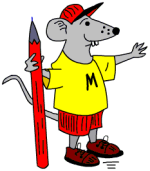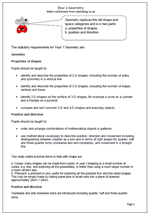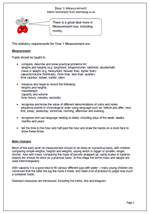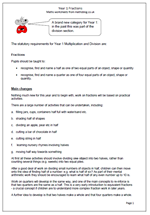## New Maths Curriculum: Year 2 Number and Place ValueThere are some tough challenges in the new Year 2 Programme of Study. Let’s begin by looking at ‘Number and place value’.
The statutory requirements for Year 2 Number and Place Value are:

Number and place value

Pupils should be taught to:

•    count in steps of 2, 3, and 5 from 0, and in tens from any number, forward or backward

•    recognise the place value of each digit in a two-digit number (tens, ones)

•    identify, represent and estimate numbers using different representations, including the number line

•    compare and order numbers from 0 up to 100; use <, > and = signs

•    read and write numbers to at least 100 in numerals and in words

•    use place value and number facts to solve problems.

Main changes
Most of this looks very similar to the old curriculum. The main difference is the introduction of the < and > signs. Remembering which is which could prove very tricky.
Writing numbers to at least 100 in words is a real challenge which quite a few children will not be able to manage by the end of the year.
A key idea in this category is partitioning numbers in different ways. For example:
24 can be split up or partitioned into 20 + 4 or 10 + 14.
This is important to understand as it helps with written addition and subtraction in later years. Also it is important to begin to understand zero as a place holder; i.e. the 1 in 100 has a value ten times the 1 in 10, with the zeros being used as place holders.
Counting in multiples of 3 is a new target, introduced to help later with understanding the concept of a third.
Also note that this new programme will not become a statutory requirement until 2015.

Year 2 Number and Place Value

## New Maths Curriculum: Year 1 GeometryGeometry replaces the old shape and space categories and is in two parts:

a. properties of shapes
b. position and direction.

The statutory requirements for Year 1 Geometry are:

Properties of shapes

Pupils should be taught to:

•    identify and describe the properties of 2-D shapes, including the number of sides and symmetry in a vertical line

•    identify and describe the properties of 3-D shapes, including the number of edges, vertices and faces

•    identify 2-D shapes on the surface of 3-D shapes, for example a circle on a cylinder and a triangle on a pyramid

•    compare and sort common 2-D and 3-D shapes and everyday objects.

Position and direction

Pupils should be taught to:

•    order and arrange combinations of mathematical objects in patterns

•    use mathematical vocabulary to describe position, direction and movement including distinguishing between rotation as a turn and in terms of right angles for quarter, half and three-quarter turns (clockwise and anti-clockwise), and movement in a straight line.

Two really useful practical items to help with shape are:

a. Cubes: many shapes can be made from cubes. In year 2 keeping to a small number of cubes, e.g. four, and exploring all the possibilities, is better than using a much larger number in a more random way.
b. Pinboard: a pinboard is very useful for exploring all the possible four and five sided shapes. This may be simply made by nailing panel pins or small nails into a piece of plywood approximately 24cm × 24cm.

Position and direction
Clockwise and anti-clockwise turns are introduced including quarter, half and three-quarter turns.

Year 1 Geometry: statutory requirements

## New Maths Curriculum: Year 1 MeasurementHere are the statutory requirements for Measurement in Year 1. There is a great deal more in Measurement now, including money and time.

Measurement

Pupils should be taught to:

•    compare, describe and solve practical problems for:
lengths and heights (e.g. long/short, longer/shorter, tall/short, double/half)
mass or weight (e.g. heavy/light, heavier than, lighter than)
capacity/volume (full/empty, more than, less than, quarter)
time (quicker, slower, earlier, later)

•    measure and begin to record the following:
lengths and heights
mass/weight
capacity and volume
time (hours, minutes, seconds)

•    recognise and know the value of different denominations of coins and notes
sequence events in chronological order using language such as: before and after, next,
first, today, yesterday, tomorrow, morning, afternoon and evening

•    recognise and use language relating to dates, including days of the week, weeks, months and years

•    tell the time to the hour and half past the hour and draw the hands on a clock face to show these times.

Main changes

Much of the early work on measurement should to be done on a practical basis, with children comparing simple lengths, heights and weights, saying which is bigger or smaller, longer, shorter. Also with mass, comparing the mass of parcels wrapped up, using scales to balance objects etc should be done on a practical basis. At this stage the terms mass and weight are used interchangeably.

With capacity it is a good idea to fill various different jugs with water – many young children are convinced that the taller the jug the more it holds, and need a lot of practice to judge how much a container holds.

Standard measures are introduced, including the metre, litre and kilogram.

Year 1 Measurement statutory requirements

## New Maths Curriculum: Year 1 FractionsFractions is a brand new category for Year 1: in the past this was part of the division section.

The statutory requirements for Year 1 Multiplication and Division are:

Fractions

Pupils should be taught to:

•    recognise, find and name a half as one of two equal parts of an object, shape or quantity

•    recognise, find and name a quarter as one of four equal parts of an object, shape or quantity.

Main changes

Nothing much new for this year and to begin with, work on fractions will be based on practical activities.

There are a large number of activities that can be undertaken, including:

a.    filling jars, cups, containers half full with water/sand etc.

c.    dividing an apple, pear etc in half

d.    cutting a bar of chocolate in half

e.    cutting string in half

f.     learning nursery rhymes involving halves

g.    moving half way towards something

At first all these activities should involve dividing one object into two halves, rather than counting several things (e.g. sweets) into two equal piles.

After a good deal of work on dividing small numbers of objects in half, children can then move onto the idea of finding half of a number: e.g. what is half of six? As part of their mental arithmetic work they should be encouraged to learn what half of any even number up to 10 is.

Work on quarters will develop in the same way, and one of the main concepts to re-inforce is that two quarters are the same as a half. This is a very early introduction to equivalent fractions – a crucial concept if children are to understand more complex fraction work in later years.

A further idea to develop is that two halves make a whole and that four quarters make a whole.

Year 1 Fractions: statutory requirements